## Saturday, May 8, 2010

### Irodov Problem 3.30

Potential difference between two points A and B , VA - VB is basically the work done by an external force in moving a unit positive charge from B to A against the electric field or alternatively it is the kinetic energy gained by a unit positive charge when it freely accelerates under the influence of the electric field from A to B. This is very similar to gravitational potential energy. If point A has a higher gravitational potential than B (i.e. A is at a higher altitude than B), then the difference in their potentials is the work done by an external force in moving a unit mass from A to B.

I shall solve this problem in two methods to show that both methods result in the same answer.Method 1 : The potential due to a charge q at a distance r, is given by. Consider an infinitesimally section of the ring at an angle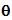that subtends an angle. The charge in this small section is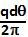. The potential due to this infinitesimally small section of the ring at a height x above the plane of the ring along the axis of the ring is given byThe potential due to the entire ring can be determined as,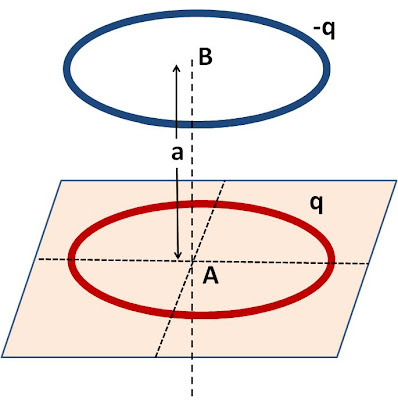Now we can solve the problem using Equation (1). The potential at point A (center of the positively charged ring) due to the positively charged ring is,The potential at point A due to the negatively charged ring is,The net potential at point A is the sum of those due to the negative and positive rings,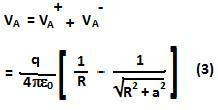Similarly the net potential at point B due to the two rings can be computed as,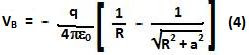The potential difference is thus given by,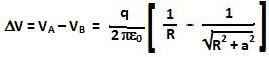Method 2 : In this method we shall use the fact that,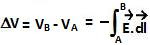From problem 3.9 (Eqn 4) we know that the electric field due to a charged ring at a height z above the plane along the axis of the positively ring is given by,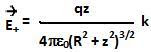The electric field due to the negatively charged ring at the same point is given by,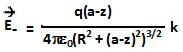The net electric field is this given by,The potential difference between points A and B can now be determined by integrating E along AB as,Clearly the potential at A is higher than that of B, since a free charge particle will move and accelerate towards point B from A gaining kinetic energy.